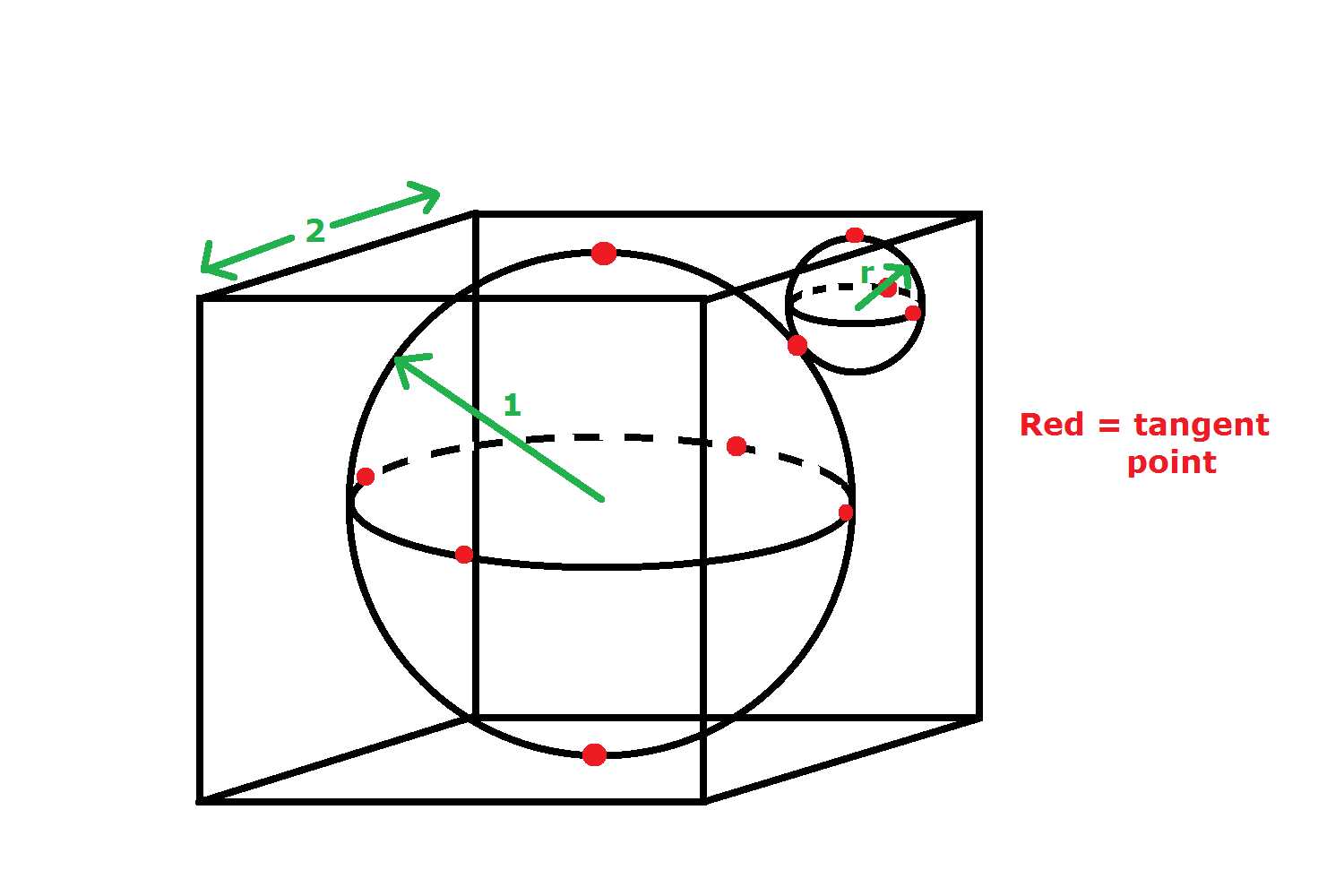# Inscribed Spheres

Geometry Level 3A unit sphere is inscribed in a cube of side length 2. A smaller sphere is inscribed in one corner of the cube, such that it lies tangent to the unit sphere and to the sides of the cube.

The radius of the smaller sphere can be written uniquely as $r = a - \sqrt{b}$, where $a$ and $b$ are positive integers and $b$ is square-free. Find $a+b.$

Inspired by abdulrahman khaled,.

×